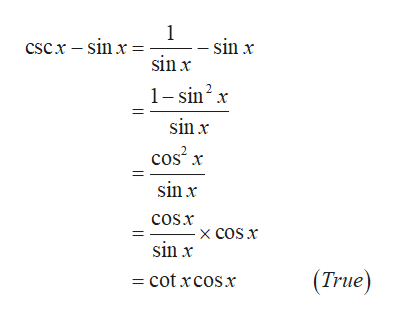# cscx-sinx=cotxcosx When using trigonometric identitities to prove if true, can you get an equivalent answer of 1=1?

Question

cscx-sinx=cotxcosx

When using trigonometric identitities to prove if true, can you get an equivalent answer of 1=1?

check_circleExpert Solution
Step 1

Proof:

...help_outlineImage Transcriptionclose1 - sin x sinx Cscx -sin x= 1-sin2 x sinx Cos x sin x cosx -X COS x sin x (True) cot xcosx fullscreen

### Want to see the full answer?

See Solution

#### Want to see this answer and more?

Solutions are written by subject experts who are available 24/7. Questions are typically answered within 1 hour*

See Solution
*Response times may vary by subject and question
Tagged in

### Other